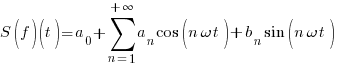# 插入数学物理公式```S(f)(t)=a_{0}+sum{n=1}{+infty}{a_{n} cos(n omega t)+b_{n} sin(n omega t)}
```

### Related Posts:

• No Related Posts